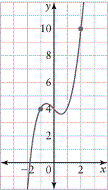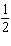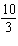Name:    1.4 Average rate of change

True/False
Indicate whether the statement is true or false.

1.

Determining the average rate of change of a function is equivalent to finding the slope of the tangent to the function.

Multiple Choice
Identify the choice that best completes the statement or answers the question.

2.

A secant drawn through the points shown on the graph has a slope ofa.c. 5 b. 2 d.3.

The average rate of change of the function y = x3 from x = 1 to x = 3 is
 a. 27 c. 13 b. 26 d. 14

4.

The average rate of change of the function y = x2x – 1 from x = –1 to x = 4 is
 a. 1 c. 2.2 b. 2 d. 11

5.

The number of people, P, at a playground after t min is given by P = t3 + 4t + 20.
The average rate of change of the number of people at the playground from 3 min to 4 min is
 a. 39 people/min c. 59 people/min b. 41 people/min d. 100 people/min